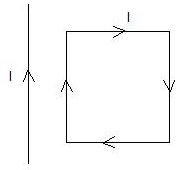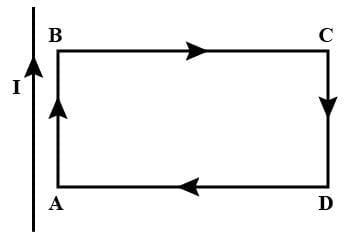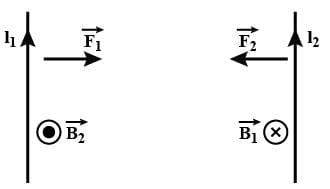NEET  >  Test: Force on Current Carrying Conductor

# Test: Force on Current Carrying Conductor

Test Description

## 10 Questions MCQ Test Physics Class 12 | Test: Force on Current Carrying Conductor

Test: Force on Current Carrying Conductor for NEET 2023 is part of Physics Class 12 preparation. The Test: Force on Current Carrying Conductor questions and answers have been prepared according to the NEET exam syllabus.The Test: Force on Current Carrying Conductor MCQs are made for NEET 2023 Exam. Find important definitions, questions, notes, meanings, examples, exercises, MCQs and online tests for Test: Force on Current Carrying Conductor below.
Solutions of Test: Force on Current Carrying Conductor questions in English are available as part of our Physics Class 12 for NEET & Test: Force on Current Carrying Conductor solutions in Hindi for Physics Class 12 course. Download more important topics, notes, lectures and mock test series for NEET Exam by signing up for free. Attempt Test: Force on Current Carrying Conductor | 10 questions in 10 minutes | Mock test for NEET preparation | Free important questions MCQ to study Physics Class 12 for NEET Exam | Download free PDF with solutions
 1 Crore+ students have signed up on EduRev. Have you?
Test: Force on Current Carrying Conductor - Question 1

### An electron and proton enter a magnetic field with equal velocities. Which one of them experiences a greater force?

Detailed Solution for Test: Force on Current Carrying Conductor - Question 1

As charges and velocities are same
F=q(V×B)
So having the same magnitude of charge and same velocity, they'll experience the same magnitude of force.

Test: Force on Current Carrying Conductor - Question 2

### Two thin long parallel wires separated by a distance b are carrying a current i ampere each. The magnitude of the force per unit length exerted by one wire on the other is

Detailed Solution for Test: Force on Current Carrying Conductor - Question 2

Given, i1​=i2​=i
∴F=μ0​i2l​/2πb
Hence, force per unit length is F=μ0​i2​/2πb

Test: Force on Current Carrying Conductor - Question 3

### The constant μo is called​

Detailed Solution for Test: Force on Current Carrying Conductor - Question 3

The permeability of free space, μ0, is numerically equal to 4π x 10-7. For air and other non-magnetic materials, the absolute permeability is the same constant. For magnetic materials, absolute permeability is not a fixed constant but varies non-linearly with the flux density.

Test: Force on Current Carrying Conductor - Question 4

A rectangular loop carrying a current I is situated near a long straight wire such that the wire is parallel to the one of the sides of the loop and is in a plane of the loop. If a steady current I is established in wire as shown in figure, the loop willDetailed Solution for Test: Force on Current Carrying Conductor - Question 4

The long straight wire and side AB  carry current in the same direction, hence will attract each other.
The long straight wire and side CD carry current in the opposite direction, hence will repel each other.
Force on side BC  will be equal and opposite to force on side DA.
Since CD  is farther from the wire than AB,  the force of attraction on  AB  will exceed the force of repulsion on CD.
Hence, there will be a net force of attraction on the loop ABCD and it will move towards the wire.Test: Force on Current Carrying Conductor - Question 5

In two current carrying conductors parallel currents________, anti parallel currents_________ .​

Detailed Solution for Test: Force on Current Carrying Conductor - Question 5

parallel currents attract from the below figure.
and similarly, in antiparallel currents repel.parallel currents attract from the below figure.
and similarly, in antiparallel currents repel.Test: Force on Current Carrying Conductor - Question 6

No force is exerted by magnetic field on a stationary

Detailed Solution for Test: Force on Current Carrying Conductor - Question 6

Electric dipole at rest can be considered as charge particles at rest. hence, no magnetic field is applied on it.

Test: Force on Current Carrying Conductor - Question 7

Along an infinitely long conductor carrying a current of 8 A we keep another conductor of length 5 m carrying a current of 3 A. Both the conductors are 10 cm apart. Find the force on small conductor.​

Detailed Solution for Test: Force on Current Carrying Conductor - Question 7

I1=3A
I2=8A
l=5m
r=0.1m
F=?
Force per unit length on the small conductor is given by:
f= μo2I1I2/4πr
Total force on the length of small conductor is
F=fl
F= μo2I1I2 l/4πr
F=4πx10-7x2x3x8x5/4π x 0.1
F=2.4x10-4N

Test: Force on Current Carrying Conductor - Question 8

We use _________ to find the direction of force when two current carrying conductors are kept parallel to each other.

Detailed Solution for Test: Force on Current Carrying Conductor - Question 8

The direction of force (motion) of a current carrying conductor in a magnetic field is given by Fleming’s left hand rule.
It states that ‘ If we hold the thumb, fore finger and middle finger of the left hand perpendicular to each other such that the fore finger points in the direction of magnetic field, the middle finger points in the direction of current, then the thumb shows the direction of force (motion) of the conductor.

Test: Force on Current Carrying Conductor - Question 9

The connecting wires of a battery of an automobile carry 200 A of current. Calculate the force per unit length between the wires if they are 50 cm long and 2 cm apart?​

Detailed Solution for Test: Force on Current Carrying Conductor - Question 9

Current in both wires, I = 200 A
Distance between the wires, r = 2 cm = 0.02 m
Length of the two wires, l = 50 cm = 0.5 m
Force between the two wires is given by the relation,
F=μoI2/2πr
where, μo = permeability of free space = 4π x 10-7 TmA-1
So, F = [4π x 10-7 x (2002)]/[2π x 0.02]
⇒   F   =  0.4 Nm-1

Test: Force on Current Carrying Conductor - Question 10

A 2 cm long copper wire having mass of 6 gm, dips in to two mercury pools to form a complete circuit. The wire is placed between the poles of a magnet which causes a field strength of 0.3 T. Find the initial upward acceleration of copper wire after 100 A of current is passed through the wire (g = 10 m/s2)

Detailed Solution for Test: Force on Current Carrying Conductor - Question 10

ma=Bil−mg
a=0.3×100×2×10−2−10×6×10−3​/ m
a= 0.6−0.06​/6×10−3
=100−10
=90ms−2

## Physics Class 12

157 videos|452 docs|213 tests
 Use Code STAYHOME200 and get INR 200 additional OFF Use Coupon Code
Information about Test: Force on Current Carrying Conductor Page
In this test you can find the Exam questions for Test: Force on Current Carrying Conductor solved & explained in the simplest way possible. Besides giving Questions and answers for Test: Force on Current Carrying Conductor, EduRev gives you an ample number of Online tests for practice

## Physics Class 12

157 videos|452 docs|213 tests

### How to Prepare for NEET

Read our guide to prepare for NEET which is created by Toppers & the best Teachers